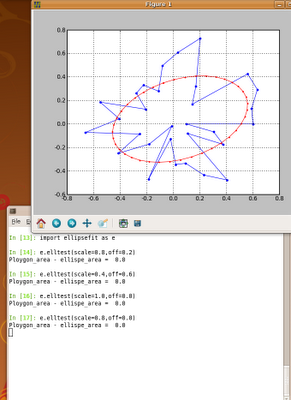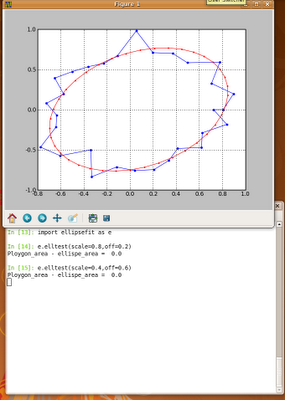## Wednesday, December 17, 2008

### Python: Ellipse fitting

The algorithm below fits an ellipse into the polygon (or set of points) in such a way that the area of the ellipse fitted is equal to the area of the polygon. `from __future__ import divisionfrom scipy import *import pylab as pdef ellipse(ra,rb,ang,x0,y0,Nb=50): '''ra - major axis length rb - minor axis length ang - angle x0,y0 - position of centre of ellipse Nb - No. of points that make an ellipse based on matlab code ellipse.m written by D.G. Long, Brigham Young University, based on the CIRCLES.m original written by Peter Blattner, Institute of Microtechnology, University of Neuchatel, Switzerland, blattner@imt.unine.ch ''' xpos,ypos=x0,y0 radm,radn=ra,rb an=ang co,si=cos(an),sin(an) the=linspace(0,2*pi,Nb) X=radm*cos(the)*co-si*radn*sin(the)+xpos Y=radm*cos(the)*si+co*radn*sin(the)+ypos return X,Y def _makexy(scale): x=reshape(array(range(scale*2)*(scale*2)),(scale*2,scale*2)) return x,x.T def _inside2(x,y,px,py): '''Check if point (x,y) inside polygon deifined by (px,py). From: http://www.ariel.com.au/a/python-point-int-poly.html ''' poly=zip(px,py) n = len(poly) inside =False p1x,p1y = poly for i in range(n+1): p2x,p2y = poly[i % n] if y > min(p1y,p2y): if y <= max(p1y,p2y): if x <= max(p1x,p2x): if p1y != p2y: xinters = (y-p1y)*(p2x-p1x)/(p2y-p1y)+p1x if p1x == p2x or x <= xinters: inside = not inside p1x,p1y = p2x,p2y return inside def _polyMask(X,Y,rs): '''Make binary mask of polygon. Values of one in 2D array pd represents points enclosed by the polygon.''' pd=zeros((rs*2,rs*2)) for i in range(pd.shape): for j in range(pd.shape): if _inside2(i,j,X,Y)==True: pd[i,j]=1.0 return pddef ellfit(X,Y,rs=50,showFig=True): '''Fit and ellipse to a polygon defined by X,Y in such a way that area of the ellispe fitted is the same as area of the polygon. Based on ellfit.pro by D.G. Long, Brigham Young University. Conditions: 1. The polygon must not be self intersecting. 2. Polygon must be closed (last point must match the first one). 3. Polygon must be centered in (0,0) 4. Polygon must be within rectangle <-1,1>x<-1,1> ''' #Xmax,Ymax=abs(X).max(),abs(Y).max() #X=X/Xmax #Y=Y/Ymax pi2=2*pi rs2=2*rs if showFig: fig = p.figure(figsize=(5,5)) ax = fig.add_subplot(111) X=X*rs+rs Y=Y*rs+rs xa,ya=_makexy(rs) pd=_polyMask(X,Y,rs) if showFig: p.plot(X,Y,"rs-",ms=15) for i in range(pd.shape): for j in range(pd.shape): if pd[i,j]==1.0: pass p.plot([i],[j],'g.',ms=10) m00=pd.sum() m10=(xa*pd).sum() m01=(ya*pd).sum() xm,ym=m10/m00, m01/m00 u20=(xa*xa*pd).sum()-xm*m10 u02=(ya*ya*pd).sum()-ym*m01 u11=(xa*ya*pd).sum()-ym*m10 tn2=2*u11/(u20-u02) ang=arctan(tn2)/2.0 if u02>u20: ang=ang-pi/2 xmean,ymean=X.mean(),Y.mean() Xr = cos(ang) * (X-xmean) - sin(ang) * (Y-ymean) +xmean Yr = sin(ang) * (X-xmean) + cos(ang) * (Y-ymean) +ymean t=_polyMask(Xr,Yr,rs) m00=t.sum() m10=(xa*t).sum() m01=(ya*t).sum() xm,ym=m10/m00, m01/m00 u20=(xa*xa*t).sum()-xm*m10 u02=(ya*ya*t).sum()-ym*m01 u11=(xa*ya*t).sum()-ym*m10 aa=((16*u20**3)/(pi2*u02))**(1/8) bb=((16*u02**3)/(pi2*u20))**(1/8) ##ellispe params a,b=max([aa,bb]),min([aa,bb]) b=t.sum()/(pi*a) ecc=sqrt(a**2-b**2) theta=ang ellArea=pi*a*b print "Ploygon_area - ellispe_area = ",round(ellArea-t.sum(),3) #print time.time()-t1 if showFig: p.grid(True) p.show() #xee,yee=b/rs*cos(0),a/rs*sin(0) #b3,a3=xee*max([Xmax,Ymax])/cos(theta),yee*min([Xmax,Ymax])/sin(theta) #print b3/a3, b/a return a/rs,b/rs,ecc,thetadef elltest(scale=0.8,off=0.2): #generate an example, random, non-self-intersecting polygon. #This is done by first generating #it in polar coordinates and than translating it #to cartesian. Theta1,R1=linspace(0,2*pi,30),rand(30)*scale+off X1,Y1=R1*cos(Theta1),R1*sin(Theta1) X1=append(X1,X1) Y1=append(Y1,Y1) p.plot(X1,Y1,".-",ms=10) a2,b2,ecc2,alpha2=ellfit(X1,Y1,showFig=False) Xe,Ye=ellipse(b2,a2,-alpha2,X1.mean(),Y1.mean(),Nb=40) p.plot(Xe,Ye,"r.-") p.grid(True) p.show() passif __name__ == '__main__': elltest()`

### Examples### Note 1

The algorithm has the following limitations:
• The polygon must not be self intersecting.
• Polygon must be closed (last point must match the first one).
• Polygon must be centered in (0,0)
• Polygon must be within rectangle <-1,1>x<-1,1>

### Note 2

This algorithm could be modified to overcome the above (Note 1) limitations. However, at the very moment I don't have time to do this. Hope this is not a problem.

### Note 3

Searching the Internet for an ellipse fitting script for Python I found fitellipse.py. It is very useful, however, sometimes I got an Exception that its not possible to fit an ellipse. The algorithm presented here seems to work good. In case the ellipse cannot be fitted try to increase rs attribute of ellfit function. The default value is 50 (rs=50).

### Note 3

This script is in ellipsefit.py.